﻿ An Implicit Response Analysis of Compressive Strength of Concrete Based on Its Ageing Periods and Cement Replacement with Bagasse Ash

### An Implicit Response Analysis of Compressive Strength of Concrete Based on Its Ageing Periods and Ce...

C. Nwoye, E. C. Chinwuko, P. C. Nwosu, N. E. Idenyi

## An Implicit Response Analysis of Compressive Strength of Concrete Based on Its Ageing Periods and Cement Replacement with Bagasse Ash

C. Nwoye1,, E. C. Chinwuko2, P. C. Nwosu3, N. E. Idenyi4

1Department of Metallurgical and Materials Engineering, Nnamdi Azikiwe University, Awka, Nigeria

2Department of Industrial and Production Engineering, Nnamdi Azikiwe University, Awka, Nigeria

3Department of Mechanical Engineering, Federal Polytechnic, Nekede, Nigeria

4Department of Industrial Physics, Ebonyi State University, Abakiliki, Nigeria

### Abstract

An implicit response analysis of the compressive strength of concrete was carried out based on its ageing periods and extent of cement replacement with bagasse ash. The response coefficient was evaluated to ascertain the viability and reliability of the compressive strength dependence of concrete on the considered input process variables. A two-factorial empirical model was derived, validated and used for the analysis and evaluation. The validity of the model; ζ = - 0.0057ϑ2 – 0.1551ϑ + 0.0002ɤ + 24.153 was rooted on the core model expression ζ - 0.0002ɤ = - 0.0057ϑ2 – 0.1551ϑ + 24.153 where both sides of the expression are correspondingly approximately equal. Regression model was used to generate results of compressive strength of concrete, and its trend of distribution was compared with that from derived model as a mean of verifying its validity relative to experimental results. The results of this verification shows similar shapes and very close alignment of curves translating into a significantly similar trend of data point’s distribution for experimental (ExD), derived model (MoD) and regression model-predicted (ReG) results. Evaluations from generated results indicated that the compressive strength per unit input of bagasse ash and ageing period as obtained from experiment, derived model & regression model were 0.3413, 0.3255 & 0.3822 N mm-2 / % and 0.1177, 0.1122 & 0.1318 N mm-2/ days, respectively. Standard errors incurred in predicting the compressive strength of concrete for each value of the bagasse ash input and an ageing period considered as obtained from experiment, derived & regression models were 0.8357, 0.6621, & 2.3973 x 10-5 % and 1.083, 0.6456 & 0.5816 %, respectively. The deviational analysis indicates that the maximum deviation of a model-predicted compressive strength of the concrete from the experimental results is less than 5%. This translated into over 95% operational confidence and response level for the derived model as well as over 0.95 response coefficient of compressive strength (of formed concrete) to the collective operational contributions of the bagasse ash input and ageing periods.

• C. Nwoye, E. C. Chinwuko, P. C. Nwosu, N. E. Idenyi. An Implicit Response Analysis of Compressive Strength of Concrete Based on Its Ageing Periods and Cement Replacement with Bagasse Ash. International Journal of Materials Lifetime. Vol. 2, No. 1, 2015, pp 13-21. http://pubs.sciepub.com/ijml/2/1/3
• Nwoye, C., et al. "An Implicit Response Analysis of Compressive Strength of Concrete Based on Its Ageing Periods and Cement Replacement with Bagasse Ash." International Journal of Materials Lifetime 2.1 (2015): 13-21.
• Nwoye, C. , Chinwuko, E. C. , Nwosu, P. C. , & Idenyi, N. E. (2015). An Implicit Response Analysis of Compressive Strength of Concrete Based on Its Ageing Periods and Cement Replacement with Bagasse Ash. International Journal of Materials Lifetime, 2(1), 13-21.
• Nwoye, C., E. C. Chinwuko, P. C. Nwosu, and N. E. Idenyi. "An Implicit Response Analysis of Compressive Strength of Concrete Based on Its Ageing Periods and Cement Replacement with Bagasse Ash." International Journal of Materials Lifetime 2, no. 1 (2015): 13-21.

 Import into BibTeX Import into EndNote Import into RefMan Import into RefWorks

12345
Prev Next

### 1. Introduction

Abrupt failures of houses and other concrete based constructions have raised an urgent need for intensive as well as extensive research and development aimed to improve the quality of cement produced and used, exploring and evaluating ways and how to produce materials that can be suitably substituted for cement, and also exploring ways of converting or incorporating industrial and agricultural wastes and cheaper locally acquired a material into cement or desirable cement substitute, respectively.

It is strongly believed that incorporation of wastes in the concrete manufacture would satisfactorily go along way, solving the problems and challenges posed by a waste management . Large quantities of natural materials are utilized by the building sectors and so there is likelihood of considerable waste recycle and upgrade .

Studies  have shown that certain cement or concrete components used in the past were derived from industrial byproducts. Other waste products can also be recycled and upgraded in concrete . It is very expedient that the newly developed material posses very satisfactory performance characteristics relative application specification if used as a building material . In evaluating new materials, it is important that traditional assessment methods have been adopted . Furthermore, the incorporation of waste into concrete should not be offensive relative to health and the environment. In addition, concrete durability should not be impaired or compromised. This stemmed from research finding  that ash has a high soluble salt content (Sugar) and therefore, impedes the strength of concrete.

The durability and the environmental impact of concrete have been reported  to be closely linked to its transport properties, which control the kinetics of the penetration of water and aggressive agents into concrete. Research  has shown that concrete diffusivity results to the movement of chemical species within the material as well as the leaching of certain chemicals

Report  has revealed that a special class of admixtures; superplasticizer, called water-reducers could lower the mix water requirement of concrete by 30% during production. The research shows that they are chemically different from normal water reducers. This class of admixtures has been characterized  as linear polymers containing sulphuric acid groups attached to the polymer backbone at regular intervals. The merit in using superplasticizers is production of flowing concrete with very high slump in the range of 175-225 mm, for use in heavily reinforced structures and in placement where adequate consolidation by vibration cannot be readily achieved. The research also revealed that placing of concrete requires much water than the cement needed for its hydration. Earlier research  has revealed that the amount of water required to obtain the desired workability and porosity (for improved ductility) could be greatly reduced by simply adding small amounts of polymeric admixtures.

It was viewed that this research will contribute to the response analysis of compressive strength of concrete produced using cheaper wastes such as bagasse ash in place of cement which is expensive. The analysis will also be based on the ageing period through which the concretes are subjected.

The aim of this work is to carry out an implicit response analysis of the compressive strength of concrete based on its ageing periods and cement replacement with bagasse ash. An empirical model would be derived, validated and used as a tool for the predictive analysis.

### 2. Materials and Methods

The bagasse used in this work was sourced from the Hausa Community in Okigwe, . The bagasse was then converted to ash by firing it at a temperature of 1200°C for 5 hrs in an electrical resistance furnace (ELOTERM).

Concrete mixtures with five different quantities of bagasse ash ranging from 0-30% were studied to determine their effect on compressive strength. The prepared mixtures were proportioned for a target cube strength of 43 N/mm2 and had a cementitious material content of 340 kg/m3, a fine aggregate content of 627 kg/m3, a coarse aggregate content of 1273kg/m3and a water cement ratio of 0.47. The setting time was determined using Humboldt’s Vicat Apparatus (HM-300). The compressive strength of the concrete was tested using a compressive machine (Model: YES-2000 with brand name NAIER). Details of the experiment and step-wise procedures used are as stated in the previous work .

#### Table 1. Variation of compressive strength of concrete with a bagasse ash input and ageing periodsDownload asVeiw tableTables index
2.1. Model Formulation

Experimental data obtained from research work  were used for this work. The computational analysis of the data  shown in Table 1, gave rise to Table 2 which indicates that;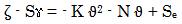(1)

Introducing the values of S, Se, K and N into equation (1) reduces it to;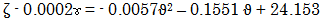(2)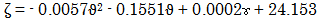(3)

Where

(ϑ) = Bagasse ash input (%)

(ɤ) = Ageing period (days)

(ζ ) = Compressive strength of concrete (N/mm2)

S = 0.0002, Se = 24.153, K = 0.0057 and N = 0.1551. S, Se, K and N are empirical constants (determined using C-NIKBRAN .

### 3. Boundary and Initial Condition

Consider a concrete forming mixture of a required consistency (in a cylindrical container) interacting with varying quantities of bagasse ash. The container’s atmosphere was not contaminated i.e (free of unwanted gases, dusts and other micro organisms). Range of bagasse ash input: 0-30 %. Range of ageing periods considered: 3 – 90 days. The range of compressive strength of concrete resulting from replacement of cement with bagasse ash: 14.59 – 24.83 N/mm2. The mass of wastes used, treatment temperature, and other process conditions are as stated in the experimental technique .

The boundary conditions are: free movement of oxygen across the cylindrical container. At the bottom of the particles, a zero gradient for the gas scalar are assumed and also for the gas phase at the top of the concrete forming mixture. The treated concrete forming mixture was stationary. The sides of the waste particles are taken to be symmetries.

### 4. Results and Discussion

4.1. Model Validation

Equation (3) is the derived model. The validity of the model was found to be rooted on equation (2) where both sides of the equation are correspondingly approximately equal. Table 2 also agrees with equation (2) following the values of ζ - 0.0002ɤ as well as 0.0057ϑ2 - 0.1551ϑ + 24.153 precisely evaluated from the experimental results in Table 1.

#### Table 2. Variation of ζ - 0.0002ɤ with - 0.0051ϑ2 - 0.1551ϑ + 24.153Download asVeiw tableTables index

In addition, the derived model was validated by comparing the compressive strength predicted by the model and that obtained from the experiment . This was done using the 4th Degree Model Validity Test Techniques (4th DMVTT); computational, graphical, statistical and deviational analysis .Download asVeiw figureFigures index
Figure 1. The coefficient of determination between the compressive strength of concrete and its ageing period as obtained from experimentDownload asVeiw figureFigures index
Figure 2. The coefficient of determination between the compressive strength of concrete and its ageing period as predicted by derived modelDownload asVeiw figureFigures index
Figure 3. The coefficient of determination between the compressive strength of concrete and bagasse ash input as obtained from experimentDownload asVeiw figureFigures index
Figure 4. The coefficient of determination between the compressive strength of concrete and bagasse ash input as predicted by derived modelDownload asVeiw figureFigures index
Figure 5. The comparison of compressive strength values of concrete (relative to its ageing period) as obtained from both experiment and derived modelDownload asVeiw figureFigures index
Figure 6. The comparison of compressive strength values of concrete (relative to bagasse ash input) as obtained from both experiment and derived modelDownload asVeiw figureFigures index
Figure 7. The comparison of compressive strength values of concrete (relative to its ageing period) as obtained from experiment , derived and regression modelsDownload asVeiw figureFigures index
Figure 8. The comparison of compressive strength values of concrete (relative to its ageing period) as obtained from experiment , derived and regression modelsDownload asVeiw figureFigures index
Figure 9. The comparison of compressive strength values of concrete (relative to bagasse ash input) as obtained from experiment , derived and regression models

4.2. Statistical Analysis

Standard Error (STEYX)

The standard errors incurred in predicting the compressive strength of produced concrete for each value of bagasse ash input and ageing periods considered as obtained from experiment, derived & regression models were 0.8357, 0.6621, & 2.3973 x 10-5 % and 1.083, 0.6456 & 0.5816 %, respectively.

Correlation (CORREL)

The correlation coefficient between compressive strength of concrete, bagasse ash input & ageing period were evaluated from the results of the derived model and experiment, considering the coefficient of determination R2 from Figure 1-Figure 4, using the expression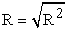(4)

The evaluated correlations using Microsoft Excel version 2003 are shown in Table 3 and Table 4. These evaluated results indicate that the derived model predictions are significantly reliable and hence valid considering its proximate agreement with results from actual experiment.

#### Table 3. The comparison of the correlations evaluated from derived model predicted and ExD results based on bagasse ash inputDownload asVeiw tableTables index

#### Table 4. The comparison of the correlations evaluated from derived model predicted and ExD results based on concrete ageing periodDownload asVeiw tableTables index
4.3. Graphical Analysis

Critical comparative graphical analysis of Figure 5 and Figure 6 show very close alignment of the curves from the experimental (ExD) and model-predicted (MoD) compressive strength. Furthermore, the degree of alignment of these curves is indicative of the proximate agreement between both experimental and model-predicted compressive strength.

4.4. Comparison of Derived Model with Standard Model

The validity of the derived model was further verified through application of the regression model (Reg) (Least Square Method using Excel version 2003) in predicting the trend of the experimental results. Comparative analyses of Figure 7-Figure 9 show similar shapes and very close alignment of curves translating into significantly similar trend of data point’s distribution for experimental (ExD), derived model (MoD) and regression model-predicted (ReG) results of compressive strength of concrete. Also, the evaluated correlations (from Figure 7-Figure 9) between compressive strength and bagasse ash input & ageing periods for results obtained from regression model gave 1.0000 & 0.9940, respectively. These values are in a proximate agreement with both experimental and derived model-predicted results. The standard errors incurred in predicting the compressive strength of concrete for each value of the bagasse ash input and the ageing period considered as obtained from regression model were 2.3973 x 10-5 and 0.5816%, respectively.

4.5. Computational Analysis

A comparative computational analysis of the experimental and model-predicted compressive strength was carried out to ascertain the degree of validity of the derived model. This was done by comparing results of the evaluated compressive strength per unit input of bagasse ash and ageing periods of concrete as obtained from experimental and derived model within bagasse ash input and concrete ageing period ranges: 0 – 30% and 3 – 90 days, respectively.

Compressive strength per unit input of bagasse ash

Compressive strength per unit input of bagasse ash

ζ / ϑ was calculated from the equation;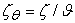(5)

Re-written as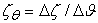(6)

Equation (6) is detailed as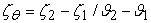(7)

Where

Δζ = Change in the compressive strength ζ2, ζ1 at two values of bagasse ash input ϑ 2 , ϑ 1.

Considering the points (0, 24.83) & (30, 14.59), (0, 24.153) & (30, 14.388) and (0, 25.2457) & (30, 13.7788) as shown in Figure 9, and are designating them as (ζ1, ϑ1) & (ζ2 ϑ2) for experimental, derived and regression models predicted results respectively, and then substituting them into equation (7), gives the slopes: - 0.3413, - 0.3255 & - 0.3822 N mm-2 / %, respectively as their corresponding compressive strength of concrete per unit input of bagasse ash.

Compressive strength per unit ageing period

Similarly, substituting into equation (8) points (3, 24.83) & (90, 14.59), (3, 24.153) & (90, 14.388) and (3, 25.2457) & (90, 13.7788) from Figure 7, as (ζ1, ɤ1) & (ζ2, ɤ2) for experimental, derived and regression models predicted results respectively also gives the slopes: - 0.1177, - 0.1122, & - 0.1318 N mm-2 / days, respectively as their corresponding compressive strength of concrete per unit ageing period. The proximity between values in each result set indicates significantly a high validity level for the derived model.

It is important to state that the actual compressive strength of concrete per unit input of bagasse ash and ageing period (as obtained from derived model and experiment) are just the magnitude of the signed value.

The associated sign preceding these values signified that the associated slope tilted to negative plane. Based on the foregoing, the compressive strength of concrete per unit input of bagasse ash and ageing period as obtained from experiment and derived model are 0.3413, 0.3255 & 0.3822 N mm-2 / % and 0.1177, 0.1122, & 0.1318 N mm-2 / days respectively.

4.6. Deviational Analysis

A comparative analysis of the compressive strengths obtained from experiment  and derived model shows low deviations on the part of the model-predicted values relative to values obtained from the experiment. This is attributed to the fact that the surface properties of the bagasse ash and the physicochemical interactions between bagasse ash and the concrete forming mixture which were believed to have played vital roles during the process  were not considered during the model formulation. This necessitated the introduction of correction factor to bring the model-predicted final setting time to those of the corresponding experimental values.

Deviation Dev, of model-predicted compressive strength from the corresponding experimental result is given by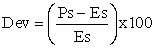(8)

Where

Es and Ps are compressive strength evaluated from experiment and derived model respectively.Download asVeiw figureFigures index
Figure 10. The variation of compressive strength with bagasse ash input and associated deviation (of model-predicted values)

The deviational analysis of Figure 10 indicates that the precise maximum deviation of model-predicted compressive strength from the experimental results is less than 5%. This translated into over 95% operational confidence and response level for the derived model as well as over 0.95 response coefficients of compressive strength (of formed concrete) to the collective operational contributions of bagasse ash input and ageing periods.Download asVeiw figureFigures index
Figure 11. The variation of ageing periods with bagasse ash input and associated deviation (of model-predicted values)

Consideration of equation (8) and critical analysis of Figure 1- Figure 4, Figure 10 and Figure 11 show that the least and highest magnitudes of deviation of the model-predicted compressive strength of concrete (from the corresponding experimental values) are + 1.3 and + 4.89%. This corresponds to compressive strengths: 24.153 and 22.0376 N/mm2; bagasse ash inputs: 0 and 10% as well as concrete ageing periods: 3 and 28 days, respectively.Download asVeiw figureFigures index
Figure 12. The variation of compressive strength with bagasse ash input and associated correction factor to model-predicted values

The correction factor took care of the negligence of operational contributions of surface properties of the bagasse ash and the physicochemical interactions between bagasse ash and the concrete forming mixture which actually played vital roles during the process . The model predicted results deviated from those of the experiment because these contributions were not considered during the model formulation. Introduction of the corresponding values of Cf from equation (10) into the model gives exactly the corresponding experimental final setting time.

Correction factor, Cf to the model-predicted results is given by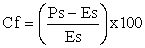(9)Download asVeiw figureFigures index
Figure 13. The variation of ageing period with bagasse ash input and associated correction factor to model-predicted values

Equations (8) and (9) indicate that the correction factor is the negative of the deviation. Furthermore, consideration of equation (9) and analysis of Figure 1- Figure 4, Figure 12 and Figure 13 indicate that the evaluated correction factors are negative of the deviation as shown in equations (8) and (9).

Figure 1- Figure 4, Figure 12 and Figure 13 also show that the least and highest correction factors (to the model-predicted compressive strength) are – 1.3 and – 4.89% which corresponds to compressive strength values: 24.153 and 22.0376 N/mm2; bagasse ash inputs: 0 and 10% as well as concrete ageing periods: 3 and 28 days, respectively.

It is important to state that the deviation of model predicted results from that of the experiment is just the magnitude of the value. The associated sign preceding the value signifies that the deviation is a deficit (negative sign) or surplus (positive sign).

### 5. Conclusion

An implicit response analysis of the compressive strength of concrete was carried out based on its ageing periods and extent of cement replacement with bagasse ash. A two-factorial empirical model was derived, validated and used for the analysis. The validity of the derived model was rooted on the core model expression ζ - 0.0002ɤ = - 0.0051ϑ2 - 0.1551ϑ + 24.153 where both sides of the expression are correspondingly approximately equal. A regression model was used to generate results of compressive strength of concrete, and its trend of distribution was compared with that from the derived model as a mean of verifying its validity relative to experimental results. The results of this verification translated into very similar shape configuration and close alignment of curves, depicting significantly similar trend of data point’s distribution for experimental (ExD), derived model (MoD) and regression model-predicted (ReG) results. Evaluations from generated results indicated that the compressive strength per unit input of bagasse ash and ageing period as obtained from experiment, derived & regression models were 0.3413, 0.3255 & 0.3822 % N mm-2 / % and 0.1177, 0.1122 & 0.1318 Nmm-2/days, respectively. Standard errors incurred in predicting the compressive strength of concrete for each value of the bagasse ash input and ageing period considered as obtained from experiment, derived model & regression model were 0.8357, 0.6621, & 2.3973 x 10-5 % and 1.083, 0.6456 & 0.5816 %, respectively. The deviational analysis indicates that the maximum deviation of model-predicted compressive strength of the concrete from the experimental results is less than 5%. This translated into over 95% operational confidence and response level for the derived model as well as over 0.95 response coefficient of compressive strength (of formed concrete) to the collective operational contributions of the bagasse ash input and ageing periods.

### References

  Cook, D.J. (1997). Sawdust ash lime cement mixes for use in masonry units, building and environment, 218-220.In article  Basher, McCabe C. C. and Long, A. E. (2005). The Influence of admixture on the properties of fresh and hardened concrete”. Journal of Scientific Industrial Research, 8: 199-214.In article  Elinwa, A. U. and Mahmood, Y. A. (2002). Ash from timber waste as cement replacement material”. Journal of Cement and Concrete Composite, 24(2): 219-222.In article View Article  Mehta, P. K. and Pirtz D. (1980). Use of bagasse ash to reduce temperature in high strength mass concrete. ACI Journal Proceedings, 81: 50-51.In article  Helmuth, R. (1987). Fly ash in cement and concrete, Portland Cement Association. Journal of Cement and Concrete Research, 30: 201-204.In article  Chatterji, A. K. (1990). Adsorption of lime and pozzolanic activity. Journal of Scientific Industrial Research, 19B: 493-494.In article  Kessler, B., Rollet, M. and Sorrentino, F. (1992). Microstructure of cement pastes as incinerators ash host. Proceedings of 1st International Symposium on cement industry solution to waste Management, Calgary. Pp: 235-251.In article  Pimienta, P., Remond, S., Rodrigues, N and, Bournazel J. P. (1999). Assessing the Properties of Mortars Containing Musical Solid Waste Incineration Fly Ash. International Congress, Creating with Concrete, University of Dundee. Pp: 319-326.In article  Remond, S,. Pimienta, p and Bentz D.P. (2002). Effect of the incorporation of municipal solid waste fly ash in cement paste and Mortars. Journal of cement and concrete research, 10:12-14.In article  Neville, A. M. (1995). Properties of concrete, 4th Edition. Pitman Publishing Comp. Ltd., New York.In article  Mielenz, R. (1996). History of chemical admixtures in concrete. Journal of Cement, Aggregate and Concrete Research, 120: 50-54.In article  Neville, A. M. and Brooks, J. (1995). Concrete technology, 3rd edition. Pearson Publishers, India.In article  Ramachandran, V. S. (2002). Superplasticizers in concrete. Journal of Cement and Concrete Research, 82: 60-62.In article  Kreijger, P. C. (1999). Plasticizers and dispersing admixtures. Proceedings of the international congress on admixtures, London. Pp: 80-83.In article  Malhotra, V. M. (1988). A global review with emphasis on durability and innovative concrete. American Concrete Institute Journal, 36: 120-130.In article  Mbadike, E. M. (2011). The Effect of Bagasse Ash on the Compression Strength of Concrete. International Journal of Natural and Applied Sciences, 7(1): 32-36.In article  Nwoye, C. I., (2008). Data Analytical Memory; C-NIKBRAN.In article PubMed  Nwoye, C. I., and Nwabanne J. T. (2013). Empirical Analysis of Methane Gas Yield Dependence on Organic Loading Rate during Microbial Treatment of Fruit Wastes in Digester. Advances in Applied Science Research 4(1): 308-318.In article